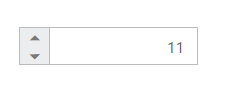RTL Support

The NumericTextBox provides RTL (Right-To-Left) support. The alignment of NumericTextBox can be changed from Left-To-Right into Right-To-Left.

Enable RTL

The following steps explain the implementation of enableRTL in NumericTextBox .

In the HTML page set the corresponding <input> elements for rendering NumericTextBox control.

• html
• <input id="numeric" type="text" ej-numerictextbox [value]="value" [enableRTL]="true"/>
• html
• import { Component, ViewEncapsulation } from '@angular/core';

@Component({
selector: 'ej-app',
templateUrl: 'src/numerictextbox/numerictextbox.component.html'
})
export class NumericTextboxComponent {
public value: number;
constructor() {
this.value = 11;
}
}

The output for NumericTextBox when enableRTL is “true” is as follows.